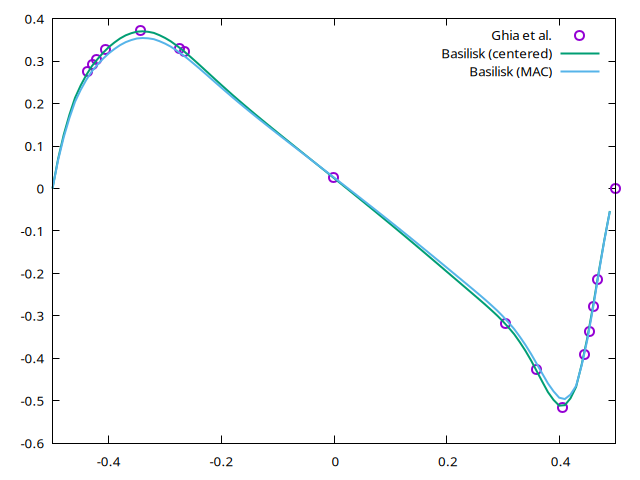# Lid-driven cavity at Re=1000

We use the multigrid implementation (rather than the default tree implementation) and either the MAC or the centered Navier–Stokes solver.

#include "grid/multigrid.h"
#if MAC
#  include "navier-stokes/mac.h"
#else
#  include "navier-stokes/centered.h"
#endif

Here we define the domain geometry: a square box of size unity centered on (0,0). We also set the viscosity and some parameters controlling the numerical scheme.

int main()
{
// coordinates of lower-left corner
origin (-0.5, -0.5);
// number of grid points
init_grid (64);
// viscosity
#if MAC
nu = 1e-3;
#else
const face vector muc[] = {1e-3,1e-3};
mu = muc;
#endif
// maximum timestep
DT = 0.1;
// CFL number
CFL = 0.8;

We then call the run() method of the Navier–Stokes solver.

  run();
}

The default boundary conditions are symmetry (i.e. slip walls). We need no-slip on three boundaries and u=1 on the top boundary i.e.

u.t[top] = dirichlet(1);

For the other no-slip boundaries this gives

u.t[bottom] = dirichlet(0);
u.t[left]   = dirichlet(0);
u.t[right]  = dirichlet(0);

For the colocated solver, imposing boundary conditions for the normal components of the (face-centered) advection velocity improves the results. Ideally, this should be done automatically by the solver.

#if !MAC
uf.n[left]   = 0;
uf.n[right]  = 0;
uf.n[top]    = 0;
uf.n[bottom] = 0;
#endif

We define an auxilliary function which computes the total kinetic energy. The function works both for face and centered discretisations of the velocity.

static double energy()
{
double se = 0.;
if (u.x.face)
foreach(reduction(+:se))
se += (sq(u.x[] + u.x[1,0]) + sq(u.y[] + u.y[0,1]))/8.*sq(Delta);
else // centered
foreach(reduction(+:se))
se += (sq(u.x[]) + sq(u.y[]))/2.*sq(Delta);
return se;
}

We add an option to restore the simulation from a previous dump.

event init (i = 0) {
#if !MAC
restore (file = "lid-restore.dump");
#endif
}

We want the simulation to stop when we are close to steady state. To do this we store the u.x field of the previous timestep in an auxilliary variable un.

scalar un[];

Every 0.1 time units we check whether u has changed by more than 10-5. If it has not, the event returns 1 which stops the simulation. We also output the evolution of the kinetic energy on standard error.

event logfile (t += 0.1; i <= 10000) {
double du = change (u.x, un);
if (i > 0 && du < 1e-5)
return 1; /* stop */
fprintf (stderr, "%f %.9f %g\n", t, energy(), du);
}

Every 100 timesteps we output a binary representation of u.x bilinearly-interpolated on an N x N grid.

event outputfile (i += 100) output_matrix (u.x, stdout, N, linear = true);

We dump a snapshot which can be used to restart the simulation.

#if !MAC
event snapshot (i = 1700)
dump (file = "dump");
#endif

This event will happen after completion of the simulation. We write in the xprof and yprof files the interpolated values of u.x and u.y along the two profiles.

event profiles (t = end)
{
FILE * fp = fopen("xprof", "w");
for (double y = -0.5; y <= 0.5; y += 0.01)
fprintf (fp, "%g %g\n", y, interpolate (u.x, 0, y));
fclose (fp);

fp = fopen("yprof", "w");
for (double x = -0.5; x <= 0.5; x += 0.01)
fprintf (fp, "%g %g\n", x, interpolate (u.y, x, 0));
fclose (fp);
}

## Results

After processing by gnuplot (i.e. using make lid/plot.png with lid.plot) we getHorizontal profile of the y-component of the velocity on the centerline of the box.